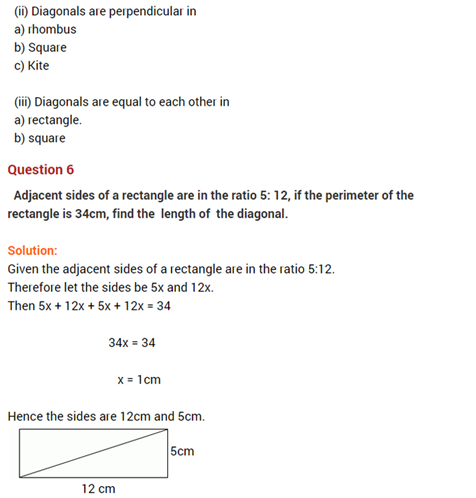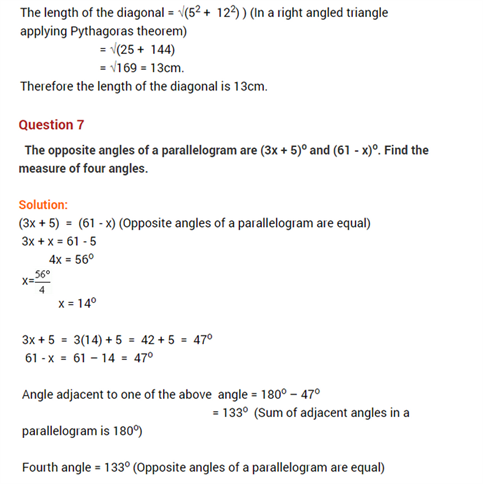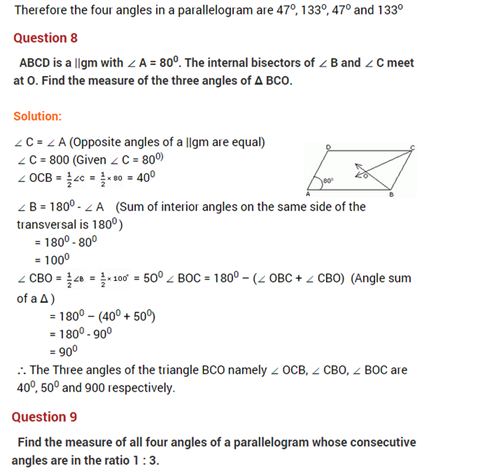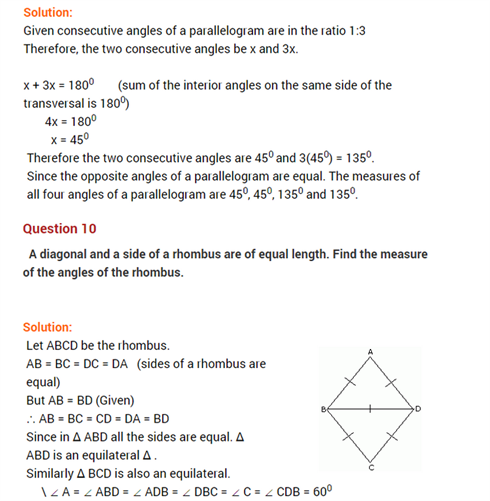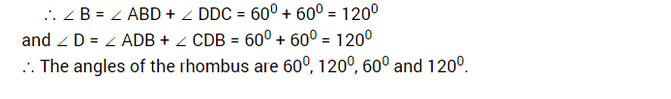# Important Questions for Maths Class 8 Chapter 3 Understanding Quadrilaterals

Important questions for Class 8 chapter 3 – Understanding quadrilaterals are given here. Students who are preparing for final exams can practice these questions to score good marks. All the questions presented here are as per NCERT curriculum or CBSE syllabus.

Understanding quadrilaterals chapter deals with different types of quadrilaterals and their properties.  We will also learn to compute the measure of any missing angle of quadrilateral. Let us see here some important questions with solutions here.

Students can also reach Important Questions for Class 8 Maths to get important questions for all the chapters here.

## Class 8 Chapter 3 Important Questions

Questions and answers are given here based on important topics of class 8 Maths Chapter 3.

Q.1: A quadrilateral has three acute angles, each measure 80°. What is the measure of the fourth angle?

Solution: Sum of the four angles of a quadrilateral = 360°

80°+80°+80°+x = 360°

x = 360 – (80 + 80 +80)

x = 360 – 240

x = 120°

Hence, the fourth angle is 120°.

Q,2: In a quadrilateral ABCD, the measure of the three angles A, B and C of the quadrilateral is 110°, 70° and 80°, respectively. Find the measure of the fourth angle.

Solution: Let,

A = 110°

B = 70°

C = 80°

D = ?

Since, we know, the sum of angles of quadrilateral ABCD is;

A + B+ C+D = 360°

110+70+80+D = 360°

260° + ∠D = 360°

D = 360° – 260°

D = 100°

Therefore, the fourth angle is 100 degrees.

Q.3: In a quadrilateral ABCD, ∠D is equal to 150° and ∠A = ∠B = ∠C. Find ∠A, ∠B and ∠C.

Solution: Given,

∠D = 150°

and

∠A = ∠B = ∠C = x

By angle sum property of quadrilateral we know,

∠A + ∠B + ∠C + ∠D = 360°

x + x +x+∠D = 360°

3x+∠D = 360°

3x = 360° – ∠D = 360° – 150°

3x = 210°

x = 70°

Hence, ∠A = ∠B = ∠C = 70°.

Q.4: The angles of a quadrilateral are in the ratio of 1:2:3:4. What is the measure of the four angles?

Solution: Given,

ratio of the angles of quadrilaterals = 1:2:3:4

Let the angles be x, 2x, 3x, 4x.

The sum of four angles of a quadrilateral is 360°.

Therefore,

x+2x+3x+4x = 360°

10x = 360

x=36

Hence,

First angle = x = 36°

Second angle = 2x = 2 × 36 = 72°

Third angle = 3x = 3 × 36 = 108°

Fourth angle = 4x = 4 × 36 = 144°

The measure of four angles is 36°. 72°, 108° and 144°.

(i) which of them have their diagonals bisecting each other?

(ii) which of them have their diagonal perpendicular to each other?

(iii) which of them have equal diagonals?

Solution:

(i) Diagonals bisect each other in:

• Parallelogram
• Rhombus
• Rectangle
• Square
• Kite

(ii) Diagonals are perpendicular in:

• Rhombus
• Square
• Kite